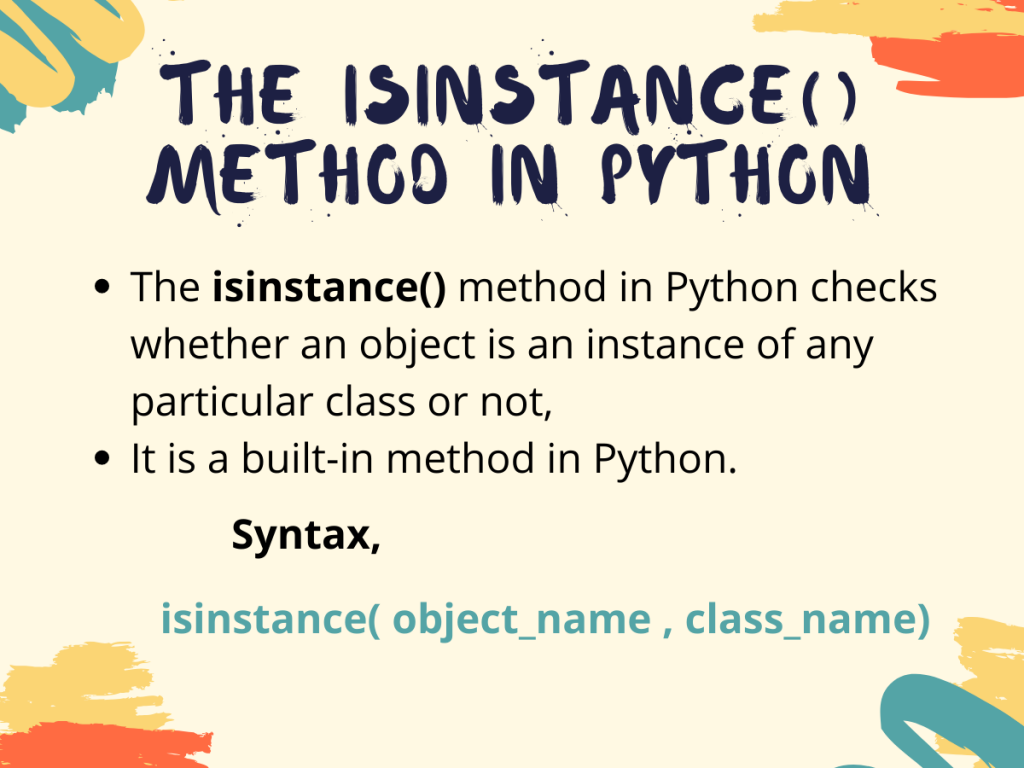# Python isinstance() Method## Introduction

In this tutorial, we’ll go over the Python isinstance() method. A user may encounter some situations where she/he needs to determine whether an object is an instance of a specific class, mainly in inheritance. Python provides an in-built method do check the same, named `isinstance()`.

Let’s get right into understanding this method.

## Python isinstance() basics

As the name suggests, the Python `isinstance()` method is an in-built method which checks whether an object is an instance of any particular class or not. Now, let us look at the syntax for using the `isinstance()` method,

Syntax,

```isinstance( object_name , class_name)
```

Here,

• `object_name` is the object for which we need to check whether it is an instance of some class or not,
• `class_name` is the class to which we need to check the object belongs to or not,
• the `isinstance()` method returns true if the object_name is an instance of the class class_name or else returns false.

## Examples of Python isinstance()

So, since now we are done with the basic definition and syntax for the Python `isinstance()` method. Let us look at some examples for better understanding of how the method works.

### 1. Using isinstance() with native classes in Python

Now, let us see what happens when we use the `isinstance()` method for native classes in python like int, float, str, list, dict, and tuple.

```i=10
f=55.55
l=[1,2,3,4,5]
d={0:"n", 1:"y"}
t=(1,2,3,4)
print("isinstance(i,int)=",isinstance(i,int))
print("isinstance(f,int)=",isinstance(f,float))
print("isinstance(s,int)=",isinstance(s,str))
print("isinstance(l,int)=",isinstance(l,list))
print("isinstance(d,int)=",isinstance(d,dict))
print("isinstance(t,int)=",isinstance(t,tuple))
```

Output:

Let’s understand what’s happening here:

• At first, we have initialized variables of different types with some values
• After that, we check whether they are instances of the corresponding classes or not. And as expected for all the checkings we get positive outputs, that is, true.

### 2. Using isinstance() with user-defined classes

Now let us create some classes and their instances to check whether we get our desired output as well as how the isinstance method works in that case.

```class dt:
dd=10
mm=2
yyyy=2002

class tm:
hr=12
min=56
sec=34
#instance creation
a=dt()
b=tm()

#check is a is instance of dt class
print("a is instance of dt?",isinstance(a,dt))
#check is a is instance of tm class
print("a is instance of tm?",isinstance(a,tm))
#check is b is instance of tm class
print("b is instance of tm?",isinstance(b,tm))
#check is b is instance of dt class
print("b is instance of dt?",isinstance(b,dt))
```

Output:

In this code:

• We create two classes `dt` and `tm `symbolizing date and time respectively
• After that is done, we create two objects of both the classes named a and b respectively
• Then we check whether the objects a and b are instances of the `dt` and `tm` classes. Using different combinations we try to explain what happens if the object is not an instance of the specified class
• As we can see, our code confirms that a and b are instances of classes dt and tm respectively.
• But the method `instance()` returns false when we try to check whether a and b are instances of tm and dt classes respectively. That is, opposite.

### 3. Using isinstance() for multiple classes

The Python `isinstance()` method also provides a measure if we want to check whether an object is an instance of any class among some given classes.

In that case, we need to pass a tuple of the required classes. The method will return `true` if the object is an instance of any class, or else returns `false`.

```class dt:
dd=10
mm=2
yyyy=2002

class tm:
hr=12
min=56
sec=34
#instance creation
a=dt()
b=tm()

#check is a is instance of dt or tm class
print("a is instance of dt or tm?",isinstance(a,(dt,tm)))
#check is b is instance of dt or tm class
print("b is instance of dt or tm?",isinstance(b,(dt,tm)))
```

Output:

```a is instance of dt or tm? True
b is instance of dt or tm? True
```

Here, we use the same classes dt and tm as we used in our previous example. But in this case, we pass a tuple of the two classes to the `isinstance()` method.

Since a and b are instances of any one of the classes in the tuple we passed, we get to see `true` in both the outputs.

## Conclusion

So, in this tutorial, we got to learn about the Python `isinstance()` method along with its uses and working. Hope this tutorial helps in understanding the concept of instances.

## References

• https://www.journaldev.com/22932/python-isinstance